# Ring

(diff) ← Older revision | Latest revision (diff) | Newer revision → (diff)

A seton which two binary algebraic operations are defined: addition and multiplication, the set being an Abelian group (the additive group of the ring) with respect to addition, and the multiplication is related to the addition by the distributive laws: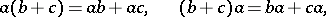where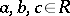. In general no restriction is imposed on multiplication, that is,is a groupoid (called the multiplicative groupoid of the ring) with respect to multiplication.

A non-empty subset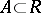is called a subring ofifitself is a ring with respect to the operations defined on, that is,must be a subgroup of the additive group ofand a subgroupoid of the multiplicative groupoid of this ring. Clearly, the ring itself and the zero subring consisting of just the zero element are subrings of a given ring. The (set-theoretic) intersection of subrings of a ring is a subring. The join of a family of subrings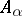,, of a ringis the intersection of all subrings that contain all. The set of all subrings of a given ring is a lattice,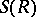, with respect to the operations of intersection and join of subrings. The set of ideals (cf. Ideal) of this ring forms a sublattice of.

Concerning the various directions in the theory of rings, see Rings and algebras; Associative rings and algebras; Non-associative rings and algebras.

In many contexts it is tacitly assumed that the ring contains a unit element, denoted by, and subrings are taken to be subrings with the same unit. In this case the set of ideals is not a sublattice of.